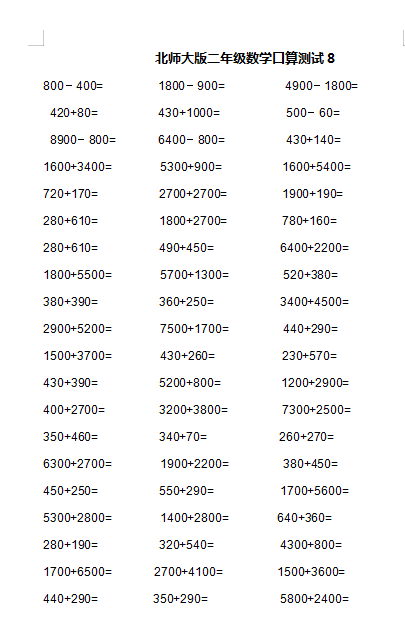800－400=         1800－900=          4900－1800=

420+80=          430+1000=           500－60=

8900－800=       6400－800=          430+140=

1600+3400=        5300+900=          1600+5400=

720+170=          2700+2700=         1900+190=

280+610=          1800+2700=         780+160=

280+610=          490+450=           6400+2200=

1800+5500=        5700+1300=         520+380=

380+390=          360+250=           3400+4500=

2022：新北师大版小学数学2年级下册口算 (1)（doc文档6电页子档下载）